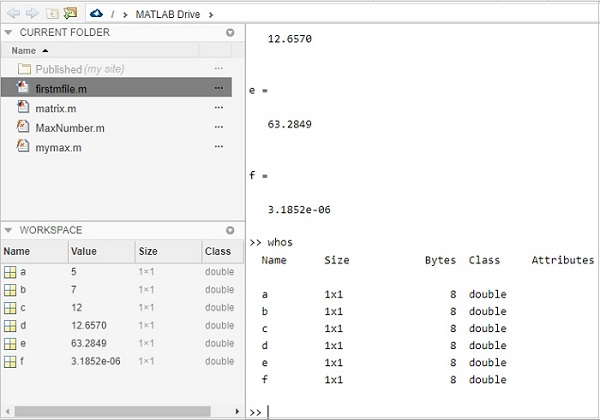# Matlab M-Files - Introduction

MATLAB allows you to write a series of commands into a file and execute the file as a complete unit, like writing a function and calling it. It is done using M-file.

M-File also called as the script file is a series of MATLAB commands that will get executed sequentially when the file is executed.

The m-file is saved with .m extension.

## Program Files

MATLAB allows writing two kinds of program files, which are as follows −

### Scripts

Script files are program files with .m extension. In these files, you write a series of commands, which you want to execute together. Scripts do not accept inputs and do not return any outputs. They operate on data in the workspace.

A script file looks as follows −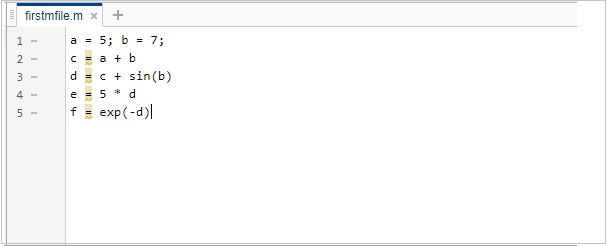### Functions

Function files are also program files with .m extension. Functions can accept inputs and return outputs. Internal variables are local to the function.

A function file looks as follows −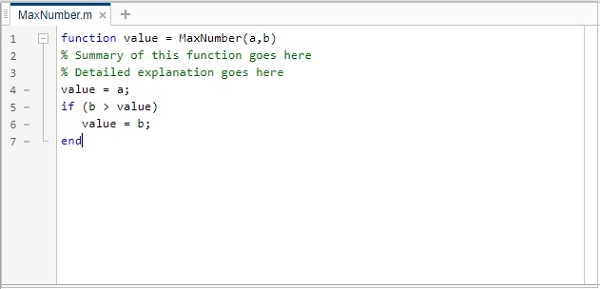Let us understand how to create and run the m-file in the next chapters.

# Matlab M-Files - Create & Save

In this chapter, we will learn how to create and save a M-file. Let us begin by understanding about creating a M-file.

## Create M-file

To create m-file, we will make use of MATLAB IDE as shown below. Here IDE refers to an integrated development environment.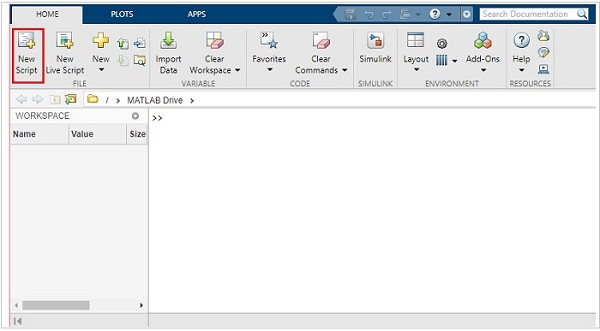Click on New Script highlighted above to open a new script file.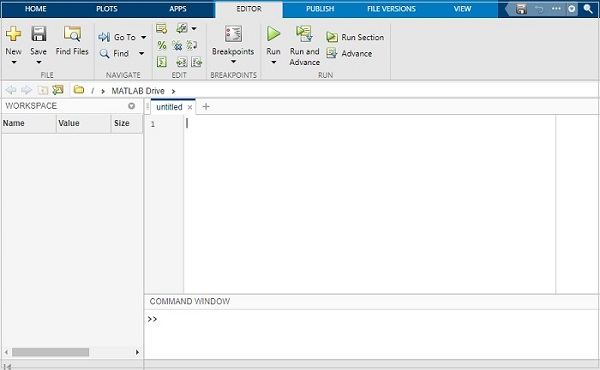## Save M-file

You will get an untitled file. Let us save the file as firstmfile.m.

Click on the save button and it will open a popup, where you can enter the name of the file.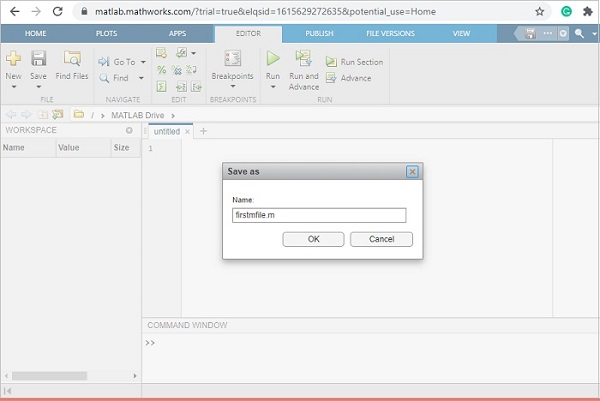Click on OK to save the file.

Now, you are free to write your commands in the file below −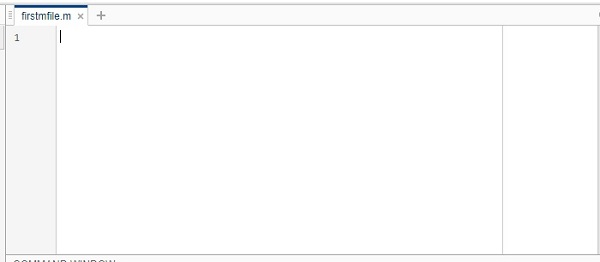# Matlab M-Files - Run

In the previous chapter, we have seen how to create and save a m-file. Now in this chapter, we will write a simple code and run the same.

Following is the code which will run inside the firstmfile.m

```a = 5; b = 7;
c = a + b
d = c + sin(b)
e = 5 * d
f = exp(-d)
```

This is how it will look inside MATLAB editor −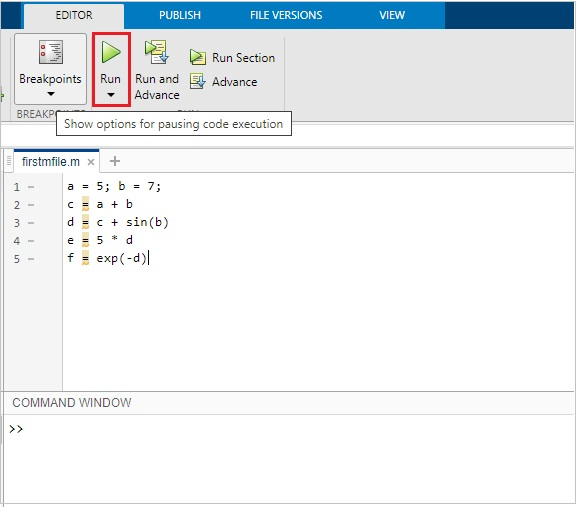Click on the Run button as highlighted above to see the result inside the command window as shown below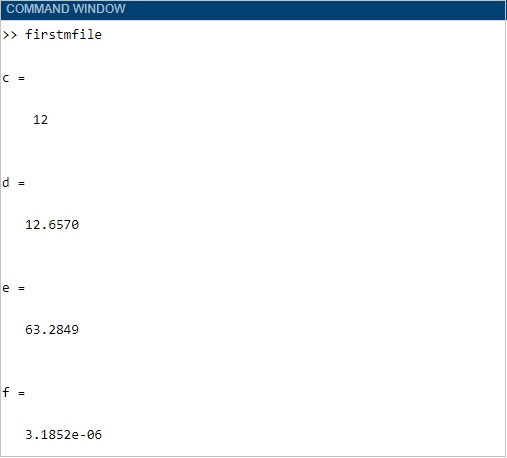# Matlab M-Files - Functions

A function is a group of statements that together perform a task. In MATLAB, functions are defined in separate files. The name of the file and name of the function should be thesame.

Functions operate on variables within their own workspace, which is also called the local workspace. These functions separate the variables from the workspace which you access at the MATLAB command prompt. This is called the base workspace.

Functions can accept more than one input arguments and may return more than one output arguments.

The syntax of a function statement is as follows −

```function [out1,out2, ..., outN] = myfun(in1,in2,in3, ..., inN)
```

Here out1, out2...outN are output variables. It can be a single variable or comma separated. The variables in1, in2, in3...inN are input variables which can be single variable or comma separated ones. The function in MATLAB starts with the keyword function as shown in the syntax.

While naming your m-file, you should take care that the file name and the function name has to match. You cannot use the name of any built-in function available in MATLAB.

Let us now create a simple function and save it as .m file and run it. In MATLAB, the IDE allows you to select what type of file you want to create as shown below −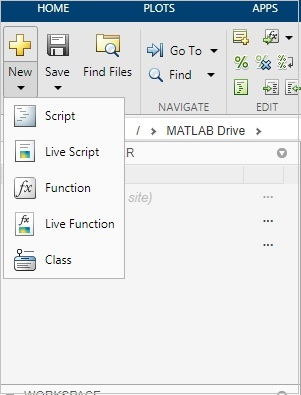Click on the Function, and it will open a new file as shown below −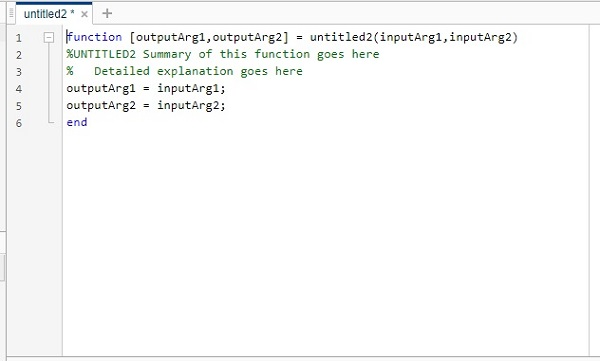Now you can update the output variables, the function name and the input variables in the above untitled file and save the file with the same name as the function name.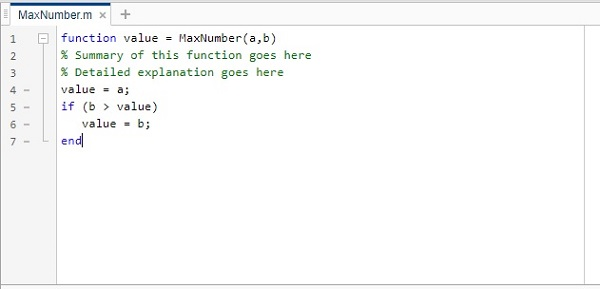The name of our function is MaxNumber() and it gives the maximum number from the input value passed.

Now let us run the function to get the output. You can call the function by using MaxNumber(100,50).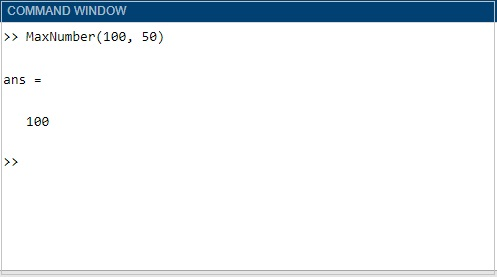# Matlab M-Files - Import Data

In this chapter, we will understand how to import data from an existing m-file in MATLAB.

Consider the m-file firstmfile.m. The contents of the file are as follows −

```a = 5; b = 7;
c = a + b
d = c + sin(b)
e = 5 * d
f = exp(-d)
```

When you execute the file, the data variables are available in the workspace, as shown below −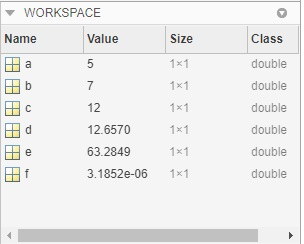Let us clear the workspace and the command prompt. Hence, we can now import the file and also check the data available.

To clear workspace, the command is clear all.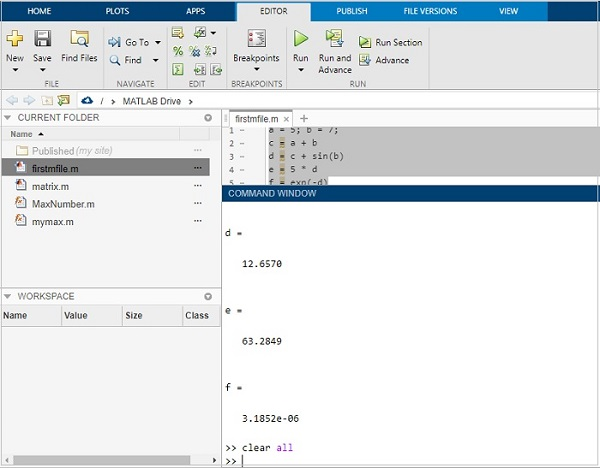The workspace is empty now.

Let us clean the command window by using clc command as shown below −We have an empty command window.

Now, type the m-file name as shown below. It will execute the code inside the file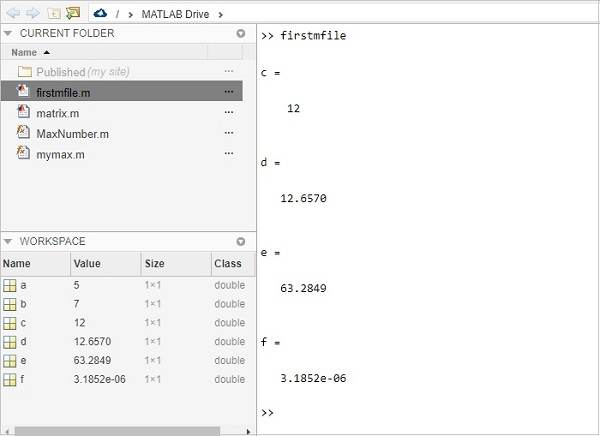The workspace is loaded with all the variables that are used inside the file.

You can also check the details of the variables by using the whos command as shown below −